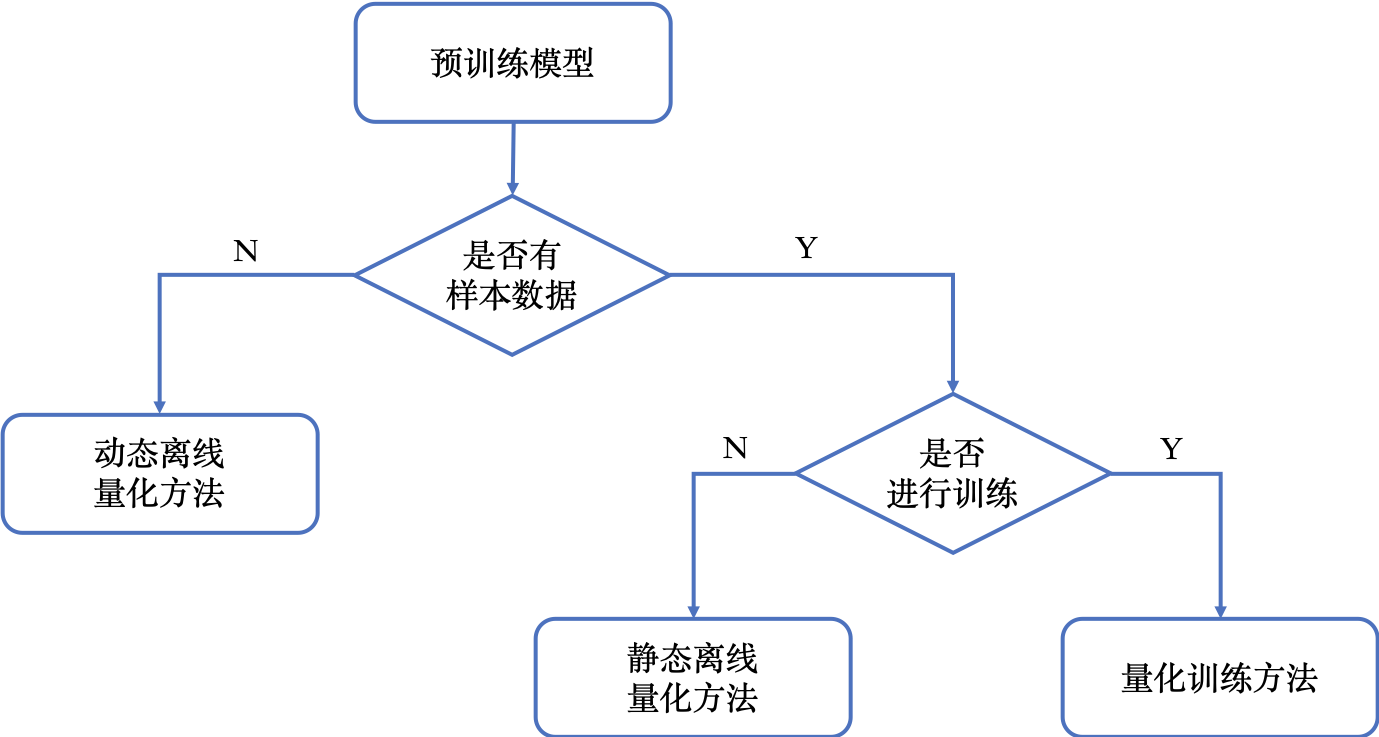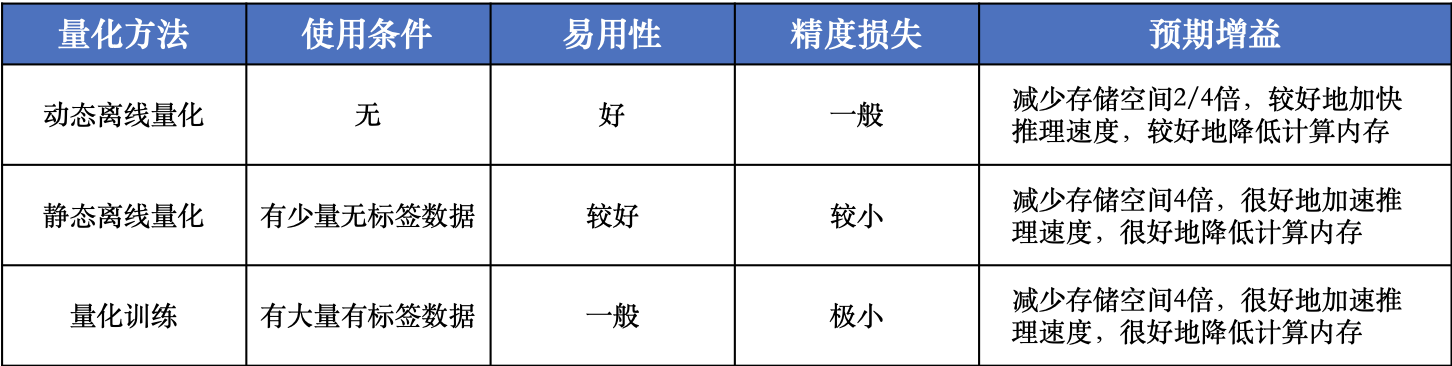# 量化¶## quant_post_dynamic¶

`paddleslim.quant.``quant_post_dynamic`(model_dir, save_model_dir, model_filename=None, params_filename=None, save_model_filename=None, save_params_filename=None, quantizable_op_type=["conv2d", "mul"], weight_bits=8, generate_test_model=False)

• 有训练好的预测模型

• 权重量化成INT16类型，模型精度不受影响，模型大小为原始的1/2
• 权重量化成INT8类型，模型精度会受到影响，模型大小为原始的1/4

• model_dir(str) - 需要量化的模型的存储路径。
• save_model_dir(str) - 量化后的模型的存储路径。
• model_filename(str, optional) - 模型文件名，如果需要量化的模型的参数存在一个文件中，则需要设置 `model_filename` 为模型文件的名称，否则设置为 `None` 即可。默认值是 `None`
• params_filename(str, optional) - 参数文件名，如果需要量化的模型的参数存在一个文件中，则需要设置 `params_filename` 为参数文件的名称，否则设置为 `None` 即可。默认值是 `None`
• save_model_filename(str, optional) - 用于保存量化模型的模型文件名，如果想让参数存在一个文件中，则需要设置 `save_model_filename` 为模型文件的名称，否则设置为 `None` 即可。默认值是 None 。
• save_params_filename(str, optional) - 用于保存模型的参数文件名，如果想让参数存在一个文件中，则需要设置 `save_params_filename` 为参数文件的名称，否则设置为 `None` 即可。默认值是 None 。
• quantizable_op_type(list[str]) - 需要量化的 op 类型列表。可选范围为 `["conv2d", "depthwise_conv2d", "mul"]` 。 默认值是 `["conv2d", "mul"]`
• weight_bits(int) - weight的量化比特位数, 可选8或者16。 默认值为8。

```import paddle

quant_post_dynamic(
model_dir='./model_path',
save_model_dir='./save_path',
model_filename='__model__',
params_filename='__params__',
save_model_filename='__model__',
save_params_filename='__params__')
```

## quant_post_static¶

`paddleslim.quant.``quant_post_static`(executor,model_dir, quantize_model_path, batch_generator=None, sample_generator=None, model_filename=None, params_filename=None, save_model_filename='__model__', save_params_filename='__params__', batch_size=16, batch_nums=None, scope=None, algo='KL', quantizable_op_type=["conv2d","depthwise_conv2d","mul"], is_full_quantize=False, weight_bits=8, activation_bits=8, activation_quantize_type='range_abs_max', weight_quantize_type='channel_wise_abs_max', optimize_model=False)

• 有训练好的预测模型
• 有少量校准数据，比如几十到几百张图片

• 减小计算量、降低计算内存、减小模型大小
• 不需要大量训练数据
• 快速产出量化模型，简单易用

• 对少部分的模型，尤其是计算量小、精简的模型，量化后精度可能会受到影响

• executor (fluid.Executor) - 执行模型的executor，可以在cpu或者gpu上执行。
• model_dir（str) - 需要量化的模型所在的文件夹。
• quantize_model_path(str) - 保存量化后的模型的路径
• batch_generator(python generator) - 读取数据样本，每次返回一个batch的数据。和 sample_generator 只能设置一个。
• sample_generator(python generator) - 读取数据样本，每次返回一个样本。
• model_filename(str, optional) - 模型文件名，如果需要量化的模型的参数存在一个文件中，则需要设置 `model_filename` 为模型文件的名称，否则设置为 `None` 即可。默认值是 `None`
• params_filename(str, optional) - 参数文件名，如果需要量化的模型的参数存在一个文件中，则需要设置 `params_filename` 为参数文件的名称，否则设置为 `None` 即可。默认值是 `None`
• save_model_filename(str) - 用于保存量化模型的模型文件名，如果想让参数存在一个文件中，则需要设置 `save_model_filename` 为模型文件的名称，否则设置为 `None` 即可。默认值是 `__model__`
• save_params_filename(str) - 用于保存模型的参数文件名，如果想让参数存在一个文件中，则需要设置 `save_params_filename` 为参数文件的名称，否则设置为 `None` 即可。默认值是 `__params__`
• batch_size(int) - 每个batch的图片数量。默认值为32 。
• batch_nums(int, optional) - 迭代次数。如果设置为 `None` ，则会一直运行到 `sample_generator` 迭代结束， 否则，迭代次数为 `batch_nums`, 也就是说参与对 `Scale` 进行校正的样本个数为 `'batch_nums' * 'batch_size'` .
• scope(fluid.Scope, optional) - 用来获取和写入 `Variable` , 如果设置为 `None` ,则使用 fluid.global_scope() . 默认值是 `None` .
• algo(str) - 量化时使用的算法名称，可为 `'KL'``'mse'`, `'hist'``'avg'`，或者 `'abs_max'` 。该参数仅针对激活值的量化，因为参数值的量化使用的方式为 `'channel_wise_abs_max'` . 当 `algo` 设置为 `'abs_max'` 时，使用校正数据的激活值的绝对值的最大值当作 `Scale` 值，当设置为 `'KL'` 时，则使用KL散度的方法来计算 `Scale` 值，当设置为 `'avg'` 时，使用校正数据激活值的最大绝对值平均数作为 `Scale` 值，当设置为 `'hist'` 时，则使用基于百分比的直方图的方法来计算 `Scale` 值，当设置为 `'mse'` 时，则使用搜索最小mse损失的方法来计算 `Scale` 值。默认值为 `'hist'`
• hist_percent(float) - `'hist'` 方法的百分位数。默认值为0.9999。
• bias_correction(bool) - 是否使用 bias correction 算法。默认值为 False 。
• quantizable_op_type(list[str]) - 需要量化的 op 类型列表。默认值为 `["conv2d", "depthwise_conv2d", "mul"]`
• is_full_quantize(bool) - 是否量化所有可支持的op类型。如果设置为False, 则按照 `'quantizable_op_type'` 的设置进行量化。如果设置为True, 则按照 量化配置`QUANT_DEQUANT_PASS_OP_TYPES + QUANT_DEQUANT_PASS_OP_TYPES` 定义的op进行量化。
• weight_bits(int) - weight的量化比特位数, 默认值为8。
• activation_bits(int) - 激活值的量化比特位数, 默认值为8。
• weight_quantize_type(str) - weight的量化方式，可选 abs_max 或者 channel_wise_abs_max ,通常情况下选 channel_wise_abs_max 模型量化精度更高。
• activation_quantize_type(str) - 激活值的量化方式, 可选 range_abs_maxmoving_average_abs_max 。设置激活量化方式不会影响计算scale的算法，只是影响在保存模型时使用哪种operator。
• optimize_model(bool) - 是否在量化之前对模型进行fuse优化。executor必须在cpu上执才可以设置该参数为True，然后会将`conv2d/depthwise_conv2d/conv2d_tranpose + batch_norm`进行fuse。

• 因为该接口会收集校正数据的所有的激活值，当校正图片比较多时，请设置 `'is_use_cache_file'` 为True, 将中间结果存储在硬盘中。另外，`'KL'` 散度的计算比较耗时。
• 目前 `Paddle-Lite` 有int8 kernel来加速的op只有 `['conv2d', 'depthwise_conv2d', 'mul']` , 其他op的int8 kernel将陆续支持。

```import paddle
use_gpu = True
place = fluid.CUDAPlace(0) if use_gpu else fluid.CPUPlace()

exe = fluid.Executor(place)
quant_post_static(
executor=exe,
model_dir='./model_path',
quantize_model_path='./save_path',
model_filename='__model__',
params_filename='__params__',
batch_size=16,
batch_nums=10)
```

## quant_aware¶

`paddleslim.quant.``quant_aware`(program, place, config, scope=None, for_test=False, weight_quantize_func=None, act_quantize_func=None, weight_preprocess_func=None, act_preprocess_func=None, optimizer_func=None, executor=None))

• program (fluid.Program) - 传入训练或测试program 。
• place(fluid.CPUPlace | fluid.CUDAPlace) - 该参数表示 `Executor` 执行所在的设备。
• config(dict) - 量化配置表。
• scope(fluid.Scope, optional) - 传入用于存储 `Variable``scope` ，需要传入 `program` 所使用的 `scope` ，一般情况下，是 fluid.global_scope() 。设置为 `None` 时将使用 fluid.global_scope() ，默认值为 `None`
• for_test(bool) - 如果 `program` 参数是一个测试 `program``for_test` 应设为True，否则设为False 。
• weight_quantize_func(function) - 自定义对权重量化的函数，该函数的输入是待量化的权重，输出是反量化之后的权重，可以快速验证此量化函数是否有效。此参数设置后，将会替代量化配置中 weight_quantize_type 定义的方法，如果此参数不设置，将继续使用 weight_quantize_type 定义的方法。默认为None。
• act_quantize_func(function) - 自定义对激活量化的函数，该函数的输入是待量化的激活，输出是反量化之后的激活，可以快速验证此量化函数是否有效。将会替代量化配置中 activation_quantize_type 定义的方法，如果此参数不设置，将继续使用 activation_quantize_type 定义的方法。默认为None.
• weight_preprocess_func(function) - 自定义在对权重做量化之前，对权重进行处理的函数。此方法的意义在于网络中的参数不一定适合于直接量化，如果对参数分布先进行处理再进行量化，或许可以提高量化精度。默认为None.
• act_preprocess_func(function) - 自定义在对激活做量化之前，对激活进行处理的函数。此方法的意义在于网络中的激活值不一定适合于直接量化，如果对激活值先进行处理再进行量化，或许可以提高量化精度。默认为None.
• optimizer_func(function) - 该参数是一个返回optimizer的函数。定义的optimizer函数将用于定义上述自定义函数中的参数的优化参数。默认为None.
• executor(fluid.Executor) - 用于初始化上述自定义函数中的变量。默认为None.

• `for_test=False` ，返回类型为 `fluid.CompiledProgram`注意，此返回值不能用于保存参数
• `for_test=True` ，返回类型为 `fluid.Program`

• 此接口会改变program 结构，并且可能增加一些persistable的变量，所以加载模型参数时请注意和相应的 program 对应。
• 此接口底层经历了 fluid.Program -> fluid.framework.IrGraph -> fluid.Program 的转变，在 `fluid.framework.IrGraph` 中没有 `Parameter` 的概念，`Variable` 只有 persistable 和not persistable的区别，所以在保存和加载参数时，请使用 `fluid.io.save_persistables``fluid.io.load_persistables` 接口。
• 由于此接口会根据 program 的结构和量化配置来对program 添加op，所以 `Paddle` 中一些通过 `fuse op` 来加速训练的策略不能使用。已知以下策略在使用量化时必须设为False ： `fuse_all_reduce_ops, sync_batch_norm`
• 如果传入的 program 中存在和任何op都没有连接的 `Variable` ，则会在量化的过程中被优化掉。

## convert¶

`paddleslim.quant.``convert`(program, place, config, scope=None, save_int8=False)

• program (fluid.Program) - 传入测试 program 。
• place(fluid.CPUPlace | fluid.CUDAPlace) - 该参数表示 `Executor` 执行所在的设备。
• config(dict) - 量化配置表。
• scope(fluid.Scope) - 传入用于存储 `Variable``scope` ，需要传入 `program` 所使用的 `scope` ，一般情况下，是 fluid.global_scope() 。设置为 `None` 时将使用 fluid.global_scope() ，默认值为 `None`
• save_int8（bool) - 是否需要返回参数为 `int8` 的 program 。该功能目前只能用于确认模型大小。默认值为 `False`

• program (fluid.Program) - freezed program，可用于保存inference model，参数为 `float32` 类型，但其数值范围可用int8表示。该模型用于预测部署。
• int8_program (fluid.Program) - freezed program，可用于保存inference model，参数为 `int8` 类型。当 `save_int8` 为False 时，不返回该值。该模型不可以用于预测部署。

```#encoding=utf8

train_program = fluid.Program()

with fluid.program_guard(train_program):
image = fluid.data(name='x', shape=[None, 1, 28, 28])
label = fluid.data(name='label', shape=[None, 1], dtype='int64')
conv = fluid.layers.conv2d(image, 32, 1)
feat = fluid.layers.fc(conv, 10, act='softmax')
cost = fluid.layers.cross_entropy(input=feat, label=label)
avg_cost = fluid.layers.mean(x=cost)

use_gpu = True
place = fluid.CUDAPlace(0) if use_gpu else fluid.CPUPlace()
exe = fluid.Executor(place)
exe.run(fluid.default_startup_program())
eval_program = train_program.clone(for_test=True)
#配置
config = {'weight_quantize_type': 'abs_max',
'activation_quantize_type': 'moving_average_abs_max'}
build_strategy = fluid.BuildStrategy()
exec_strategy = fluid.ExecutionStrategy()
#调用api
quant_train_program = quant.quant_aware(train_program, place, config, for_test=False)
quant_eval_program = quant.quant_aware(eval_program, place, config, for_test=True)
#关闭策略
build_strategy.fuse_all_reduce_ops = False
build_strategy.sync_batch_norm = False
quant_train_program = quant_train_program.with_data_parallel(
loss_name=avg_cost.name,
build_strategy=build_strategy,
exec_strategy=exec_strategy)

inference_prog = quant.convert(quant_eval_program, place, config)
```

## 量化训练方法的参数配置¶

```TENSORRT_OP_TYPES = [
'leaky_relu'
]
TRANSFORM_PASS_OP_TYPES = ['conv2d', 'depthwise_conv2d', 'mul', 'conv2d_transpose']

QUANT_DEQUANT_PASS_OP_TYPES = [
"pool2d", "elementwise_add", "concat", "softmax", "argmax", "transpose",
"equal", "gather", "greater_equal", "greater_than", "less_equal",
"less_than", "mean", "not_equal", "reshape", "reshape2",
"bilinear_interp", "nearest_interp", "trilinear_interp", "slice",
"squeeze", "elementwise_sub", "relu", "relu6", "leaky_relu", "tanh", "swish"
]

_quant_config_default = {
# weight quantize type, default is 'channel_wise_abs_max'
'weight_quantize_type': 'channel_wise_abs_max',
# activation quantize type, default is 'moving_average_abs_max'
'activation_quantize_type': 'moving_average_abs_max',
# weight quantize bit num, default is 8
'weight_bits': 8,
# activation quantize bit num, default is 8
'activation_bits': 8,
# ops of name_scope in not_quant_pattern list, will not be quantized
'not_quant_pattern': ['skip_quant'],
# ops of type in quantize_op_types, will be quantized
'quantize_op_types': ['conv2d', 'depthwise_conv2d', 'mul'],
# data type after quantization, such as 'uint8', 'int8', etc. default is 'int8'
'dtype': 'int8',
# window size for 'range_abs_max' quantization. defaulf is 10000
'window_size': 10000,
# The decay coefficient of moving average, default is 0.9
'moving_rate': 0.9,
# if True, 'quantize_op_types' will be TENSORRT_OP_TYPES
'for_tensorrt': False,
# if True, 'quantoze_op_types' will be TRANSFORM_PASS_OP_TYPES + QUANT_DEQUANT_PASS_OP_TYPES
'is_full_quantize': False
}
```

• weight_quantize_type(str) - 参数量化方式。可选 `'abs_max'` , `'channel_wise_abs_max'` , `'range_abs_max'` , `'moving_average_abs_max'` 。如果使用 `TensorRT` 加载量化后的模型来预测，请使用 `'channel_wise_abs_max'` 。 默认 `'channel_wise_abs_max'`
• activation_quantize_type(str) - 激活量化方式，可选 `'abs_max'` , `'range_abs_max'` , `'moving_average_abs_max'` 。如果使用 `TensorRT` 加载量化后的模型来预测，请使用 `'range_abs_max', 'moving_average_abs_max'` 。，默认 `'moving_average_abs_max'`
• weight_bits(int) - 参数量化bit数，默认8, 可选1-8，推荐设为8，因为量化后的数据类型是 `int8`
• activation_bits(int) - 激活量化bit数，默认8，可选1-8，推荐设为8，因为量化后的数据类型是 `int8`
• not_quant_pattern(str | list[str]) - 所有 `name_scope` 包含 `'not_quant_pattern'` 字符串的 op ，都不量化, 设置方式请参考 fluid.name_scope
• quantize_op_types(list[str]) - 需要进行量化的 op 类型，可选的op类型为 `TRANSFORM_PASS_OP_TYPES + QUANT_DEQUANT_PASS_OP_TYPES`
• dtype(int8) - 量化后的参数类型，默认 `int8` , 目前仅支持 `int8`
• window_size(int) - `'range_abs_max'` 量化方式的 `window size` ，默认10000。
• moving_rate(int) - `'moving_average_abs_max'` 量化方式的衰减系数，默认 0.9。
• for_tensorrt(bool) - 量化后的模型是否使用 `TensorRT` 进行预测。如果是的话，量化op类型为： `TENSORRT_OP_TYPES` 。默认值为False.
• is_full_quantize(bool) - 是否量化所有可支持op类型。可量化op为 `TRANSFORM_PASS_OP_TYPES + QUANT_DEQUANT_PASS_OP_TYPES` 。 默认值为False.

## quant_embedding¶

`paddleslim.quant.``quant_embedding`(program, place, config=None, scope=None)

`Embedding` 参数进行量化。

• program(fluid.Program) - 需要量化的program
• scope(fluid.Scope, optional) - 用来获取和写入 `Variable`, 如果设置为 `None`,则使用 fluid.global_scope() .
• place(fluid.CPUPlace | fluid.CUDAPlace) - 运行program的设备
• config(dict, optional) - 定义量化的配置。可以配置的参数有 ‘quantize_op_types’, 指定需要量化的op，如果不指定，则设为 [‘lookup_table’, ‘fused_embedding_seq_pool’, ‘pyramid_hash’] ,目前仅支持这三种op。对于每个op，可指定以下配置： `'quantize_type'` (str, optional): 量化的类型，目前支持的类型是 `'abs_max', 'log'`, 默认值是 `'abs_max'``'quantize_bits'` （int, optional): 量化的bit数，目前支持的bit数为8。默认值是8. `'dtype'` (str, optional): 量化之后的数据类型， 目前支持的是 `'int8'`. 默认值是 `int8` 。举个配置例子，可以是 {‘quantize_op_types’: [‘lookup_table’], ‘lookup_table’: {‘quantize_type’: ‘abs_max’}}

fluid.Program

```import paddle

train_program = fluid.Program()
with fluid.program_guard(train_program):
input_word = fluid.data(name="input_word", shape=[None, 1], dtype='int64')
input_emb = fluid.embedding(
input=input_word,
is_sparse=False,
size=[100, 128],
param_attr=fluid.ParamAttr(name='emb',
initializer=fluid.initializer.Uniform(-0.005, 0.005)))

infer_program = train_program.clone(for_test=True)

use_gpu = True
place = fluid.CUDAPlace(0) if use_gpu else fluid.CPUPlace()
exe = fluid.Executor(place)
exe.run(fluid.default_startup_program())

config = {
'quantize_op_types': ['lookup_table'],
'lookup_table': {
'quantize_type': 'abs_max'
}
}
quant_program = quant.quant_embedding(infer_program, place, config)
```